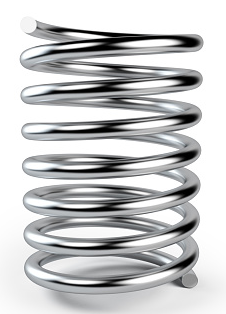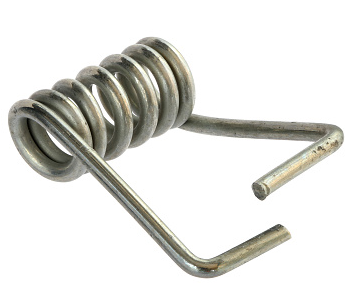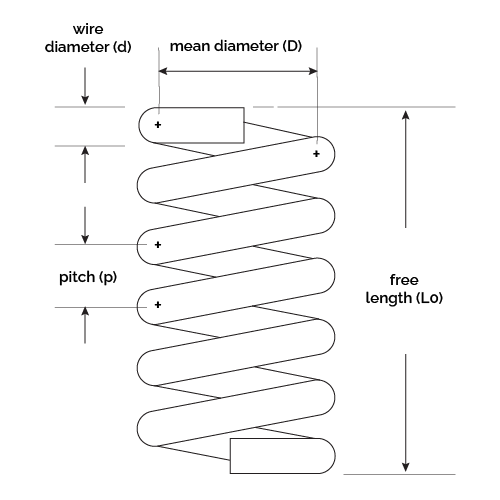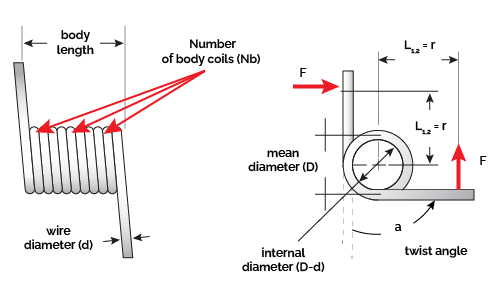# Spring Calculator

Created by Arturo Barrantes
Reviewed by Steven Wooding
Based on research by
Harold Carlson Spring Designer's Handbook (1978)
Last updated: Jan 02, 2023

The handy spring calculator expresses the relationship between the force or torque applied to a spring and its deformation. This article will show you how to use this Omni Calculator spring compression tool. Also, we will cover what the spring compression formula is and how to calculate the spring constant for different configurations. Keep reading to understand this amazing mechanism that has been used for a long time.

## What is a spring?

A spring is a mechanical device that stores energy while deforming its shape when a force or torque is applied. If you want to know specifically which type of energy, check the elastic potential energy calculator.

There are several types of springs; however, in this spring calculator, we will cover the three main types of springs. Its categorization depends on the direction of the external force or if torque is being applied. Such types are:

• Compression springs: Springs that are designed to work under a compression force.
• Expansion springs: Springs that are designed to work under a traction force.

In both cases above, the spring compression formula applies but in different directions, as you will see in further sections. Also, both follow the same behavior as the one explained in Hooke's law calculator.• Torsion springs: These are springs that experience momentum due to a force that is being applied outside of the center of gravity of the spring, specifically in one of the spring legs. Such force would make the spring rotate if we did not fix the other leg. Since the spring does not rotate, it deforms because of the torsional force, and it stores energy like the other springs.## How to calculate spring force in a compression / expansion spring?

Although any spring follows the same principle as the energy conversion calculator, expansion/compression springs and torsion springs have different formulas. In this section, we will cover the equation for the force of a spring:

$F = - k_{\rm tc} \times \Delta x,$

where:

• $F$Force – represents the force that reacts to the compression or compression of a spring, measured in Newtons;
• $k_{\rm tc}$Spring constant for traction or compression – indicates the linear relation between the change in spring length and the force applied. It is measured in Newton/meter; and
• $\Delta x$Spring change in length due to the force applied. It is measured in meters (positive for elongation and negative for compression).

Furthermore, if we want to buy some springs, we need to specify more detailed information. You might have already guessed that the spring constant should also be related to its shape. Indeed, the compression of a spring that is thicker than another is much more complicated if we assume we are dealing with the same material.

The following equation relates material properties and geometrical shape in an equation that is called: the spring rate formula:

$k_{\rm tc} = \frac{G \times d^4} {8 \times (D^3) \times N_{\rm a}},$

where:

• $G$Shear modulus - Also known as modulus of rigidity, it measures the elastic shear stiffness of a material. You will typically find its values expressed in GPa.
• $d$Wire diameter - Represents wire thickness.
• $D$Mean diameter - The mean diameter indicates the size between the outer and inside diameter.
• $N_{\rm a}$Number of active coils - Indicates the number of coils that are working when the spring is under load.

See the next picture for further details:Give a check to the Free length $L_0$ and Spring pitch ($p$) on the image above since we are going to cover them in further detail below.

## How to design a expansion / compression spring?

Our excellent spring calculator includes all the details you would need for designing a spring; however, there are three design parameters we have not explored:

• Spring index ($C$): Mathematically, it is the relation between the mean spring diameter $D$ and the wire thickness $d$: $C = D/d$. Manufacturers and spring users utilize it to define the manufacturability of a spring. Usually, a spring with a spring index between 5 and 10 represents an easy/not costly spring to manufacture.

• Free length value of a spring ($L_0$) refers to the spring length with no load.

• Spring pitch ($p$): Refers to the space between two adjacent coils and is directly related to the free length value ($L_0$) of a spring.

There is a last design parameter but similarly to the pitch, it only matters to expansion/contraction springs:

• Spring end type: This property refers to how are the external faces of a spring. We have included four types: grounded, squared, plain, and squared & ground.## How do you calculate coil spring momentum in a torsion spring?

We have to do a further step in explaining "how to calculate spring force" in the case of a torsion spring.

$M = k_{\rm torsion} \times \alpha$

where:

• $M$Torque – Torque represents the rotational equivalent of a linear force. It is expressed in Newton meters.
• $k_{\rm torsion}$Spring constant for torsion – Indicates the relation between the spring twist and the momentum applied. It is measured in Newton meters per radian.
• $\alpha$Twist angle – Represents the achieved angle while twisting the spring. It is expressed in radians.

Once we get the momentum, we need the length of the distance of lever arm $r$ (distance between the force applied and the center of rotation of the spring) and by solving the following equation:

$M= F \times r$

We get the force ($F$) applied to a torsion spring.

Finally, we will cover the design spring equation:

$k_{\rm torsion} = \frac{d^4 \times E}{64 \times D \times N_{\rm a}}$

where:

• $k_{\rm torsion}$— Torsional spring stiffness, in N·m/rad;
• $E$Elasticity modulus – Represents the material resistance to being deformed elastically. Usually expressed in GPa.
• $d$ — Wire diameter, in m;
• $D$ — Mean coil diameter, in m; and
• $N_{\rm a}$ — Equivalent number of active turns (dimensionless).

See the next picture for further details.We obtain $N_{\rm a}$ with the number of body coils $N_{\rm b}$) and the lengths ($l_1$ and $l_2$, expressed in meters) of the ends where the torsional force is applied:

$N_{\rm a} = N_{\rm b} + \frac{l_1+l_2}{3\times \pi \times D}$

Note from the picture above that at least one of $l_1$ and $l_2$ will be the length of the distance of the lever arm $r$ because it represents where the torsional force is applied. The other could be the same but also could vary.

## How to calculate spring constant and design one? – Real life example

Let's assume you need an expansion spring for a 20-kilogram load. You need it to have a maximum extension of an extra 20 cm, be made of stainless material, and have a maximum outer diameter of 4 cm. The parameters we need to obtain are the number of active coils and the spring pitch.

Try the spring calculator for different spring indexes ($C$) and free length values ($L_0$). In this example, we will consider 9 and 20 cm, respectively.

1. Let's define the information we have. According to the spring formula, we have $F = 196.2\ \rm N$, and $x=0.2\ \rm m$. That means we have a spring coefficient equal to $k_{\rm tc} = 981\ \rm N/m$.

2. Let's choose a spring index of 9 because of its manufacturability and a maximum outer diameter of 0.04 m. Such spring index indicates a mean diameter $D$ nine times spring wire thickness $d$. Considering the outer diameter, we get a spring thickness of 4 mm. Hence a mean diameter of 36 mm.

3. Now, we proceed to get the number of active coils by using the spring rate formula:

$\qquad k_{\rm tc} = \frac{G \times d^4}{8 \times D^3 \times N_{\rm a}}$

Solving the equation considering $G = 77\ \rm GPa$ returns 53.83 ~ 54 active coils.

1. Finally, if we need it with plain ends and a free length of 20 cm, we get a pitch of 3.64 mm.

## FAQ

### How to calculate spring constant?

In compression springs, we have an experimental way of determining the spring constant:

1. Get a known weight. Let's say a kilogram (1 kg). Now convert it to force: 1 kg × 9.81 m/s² equals 98.1 Newtons.

2. Measure the free length of the spring (before applying any load).

3. Put the weight over the spring. Measure the new spring length. Get the difference (x), also called displacement.

4. Divide the force by the displacement. The result is the spring constant.

### How to calculate the spring constant in a torsion spring?

You can use the Omni Calculator tool spring calculator or do as follows:

1. Find out the applied torque. Remember that torque equals force multiplied by the distance of the lever arm.

2. Measure how many degrees the spring has been twisted.

3. Divide the torque by the twist angle. The result is the spring constant for a torsion spring, expressed in N·m/°.

### How to calculate spring force?

You can use the Omni Calculator tool compression spring calculator or follow the next steps:

1. Find out the spring constant, K. For it, you will need the number of active coils (Na), spring mean diameter (D), shear modulus (G), and wire diameter (d).

2. Once you have found the values above, solve the following equation:

K = (G × d4)/(8 × D3 × Na)

3. The previous step provided the spring constant: K. The last step is to simulate a spring displacement (x).

4. Multiply the spring displacement (x) by the spring coefficient K. The result represents the spring force.

### What is the spring constant at 2 in of compression and 10 lbf?

5 lbf/in. You can verify it using the Omni Calculator tool spring rate calculator or do as follows:

1. Divide the force by the change in spring length: 10 lbf by 2 in.
2. The result is 5 lbf/in.
Arturo Barrantes
⚙️ Choose the proper settings for you
Traction/compression
Spring design parameters
Spring index (C)
Outer diameter (OD)
in
Mean diameter (D)
in
Wire diameter (d)
in
Traction compression spring
Force applied to the spring (F)
lbf
Extension/compression Δx
in
Spring constant (k)
N/m
Modulus of rigidity (G)
GPa
Choice spring end:
Plain end
Free length for plain ends
mm
Spring design results
Number of active coils (Na)
Pitch
in
People also viewed…

### Grams to cups

The grams to cups calculator converts between cups and grams. You can choose between 20 different popular kitchen ingredients or directly type in the product density.

### Humans vs vampires

Vampire apocalypse calculator shows what would happen if vampires were among us using the predator - prey model.

### MOSFET threshold voltage

Learn how to calculate the most critical parameter of a MOSFET with our MOSFET threshold voltage calculator.

### Power factor

The power factor calculator helps you to describe the relationship between the real, reactive, and apparent power in an AC circuit.